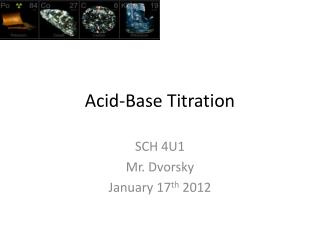DownloadDownload PresentationAcid-Base Titration

# Acid-Base Titration

Télécharger la présentation## Acid-Base Titration

- - - - - - - - - - - - - - - - - - - - - - - - - - - E N D - - - - - - - - - - - - - - - - - - - - - - - - - - -
##### Presentation Transcript

1. Acid-Base Titration SCH 4U1 Mr. Dvorsky January 17th 2012

2. Endpoint Indicators • When performing an acid-base titration, you use an indicator to determine the endpoint. • The endpoint of a titration is the pH at which the indicator changes colour.

3. Endpoint Indicators • There are many endpoint indicators, and together they cover the whole range of possible pH values.

4. Endpoint Indicators • Indicators are weak acids. The acid is one colour and the conjugate base is another colour. You can write general equilibrium equation for indicators. • E.g. HInd represents the acid form of the indictator and Ind- the conjugate base, Hind(aq) + H2O(l)  H3O+(aq) + Ind-(aq) Colour 1 Colour 2

5. Strong Acid-Base Titration • Sometimes a titration is monitored using a pH meter. With a pH meter you can monitor the titration reaction as it proceeds instead of merely estimating the pH with an indicator. • Consider a titration reaction between a strong acid and strong base that is monitored using a pH meter….

6. Strong Acid-Base Titration • A few drops of indicator are added to the solution that will change colour near the equivalence point. • The equivalence point is the point in a titration at which the amont (in moles) of the substance added is stoichiometrically equivalent to the amount (in moles) present in the original test solution, or the point at which the acid and base have completely reacted. The endpoint of the titration is when the indicator changes colour.

7. Strong Acid-Base Titration • Consider a titration in which 40.00 ml solution of a 0.1000 mol/L hydrochloric acid, HCl (aq) is titrated with 0.1000 mol/L sodium hydroxide, NaOH(aq), as represented by the equation below: HCl (aq) + NaOH(aq)  NaCl(aq) + H2O (l) The portion of the equation that involves H+ and OH- ions is shown below: H+(aq) + OH-(aq)  H2O(l)

8. Strong Acid-Base Titration • The volume of sodium hydroxide that is added to the flask and the pH of the resulting solution are plotted on the graph below: This is called a titration curve. Notice the pH of the solution increases slowly at first. The low pH indicates high [H+] from the strong acid. As more NaOH is added, the [OH-] increases. Then near the equivalence point, the slope of the curve is almost vertical. In S.A + S.B titration, the concentration of H+ and OH- is very small near the equivalence point. A small drop of NaOH can cause a large increase in pH near this point. As more NaOH is added, the pH slowly increases as you go beyond the equivalence point.

9. Weak Acid-Strong Base Titration • Weak acid only ionizes partially • This fact must be considered in calculations The dotted line at the bottom is from the strong acid-base curve, shown for easy comparison This graph shows the titration of the weak acid CH3CH2COOH(aq) with the strong base NaOH(aq). See reaction on board:

10. Weak Acid-Strong Base Titration • initial pH is higher for weak acid. –ionizes partially so less H+present. Notice that there is a buffer region: Khan academy clip explains this very well. Notice the pH of the equivalence point. It is greater than 7.00. The weak acid anion acts as a base Beyond the equivalence point, the pH of the solution increases as excess base is added.